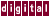## 7.3.1 Induction Variable Recognition

Induction variables are integer or floating point variables that are incremented or decremented the same amount for each `for` -loop iteration. Recognizing their relationship to the loop control variables often lifts data dependence restrictions and permits further optimization, as shown in the following example:

```for ( i=0; i<n; i++ ) {
a[j] = b[k];
j++;
k+=2;
}
```

Becomes:

```_Kii2 = k;
_Kii1 = j;
for ( i = 0; i<n; i++ ) {
a[_Kii1+i] = b[_Kii2+i*2];
}
```

Previous Page Next Page Contents Index
Command-Line Qualifiers